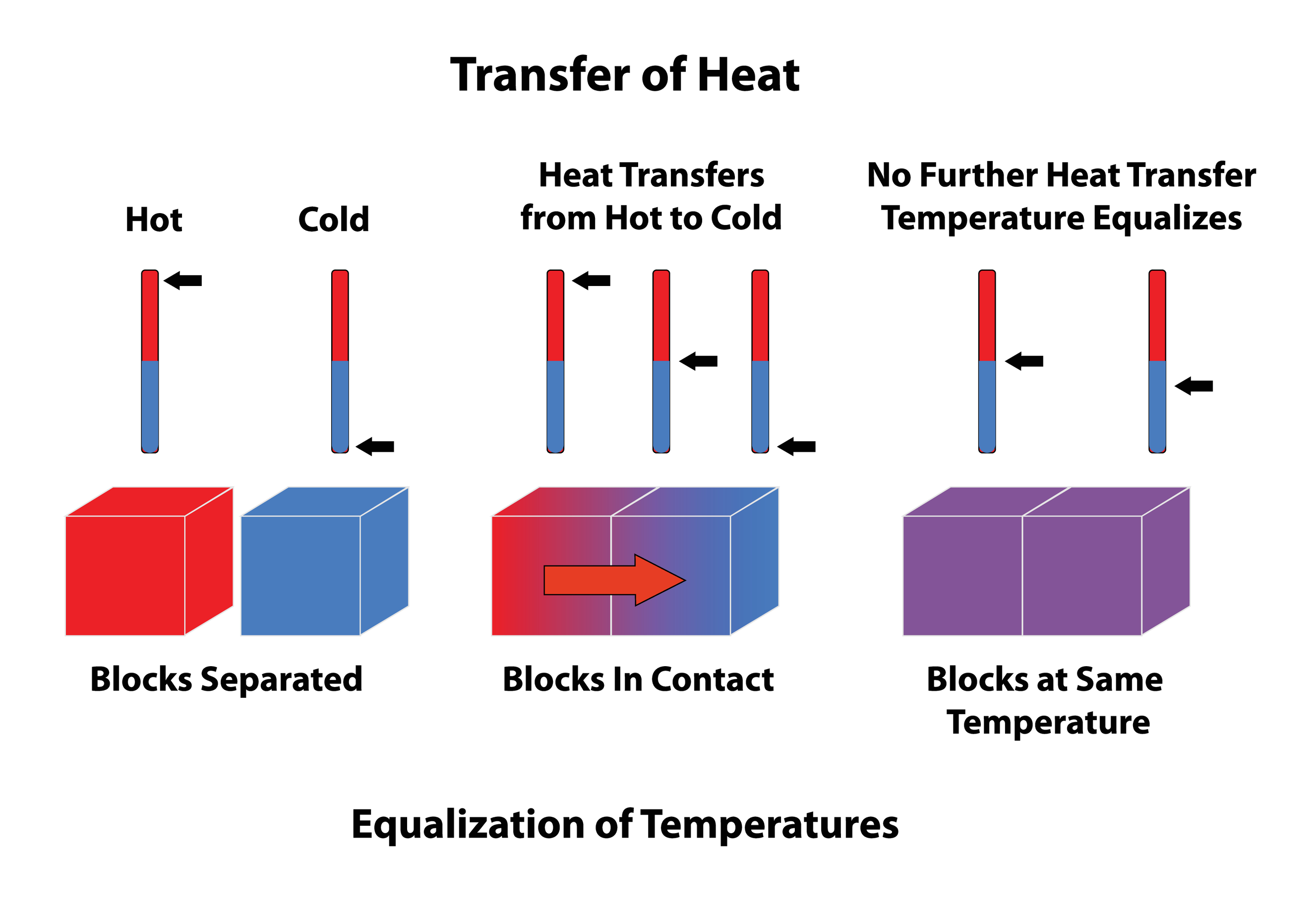×## Introduction

While we know that thermodynamic variables such as pressure, volume, and temperature affect the expansion and compression of gases, properties like the internal energy, enthalpy, and entropy also play a role. Enthalpy and energy are related concepts and in an ideal gas, the internal energy and enthalpy depend solely on temperature of the system. In fact, enthalpy changes at constant pressure involve heat supplied by or to the system, and the change in enthalpy for a reaction equals the heat absorbed or released. In accordance with the law of conservation of energy, heat flows from hotter body to colder body. Enthalpy has the same unit as energy and depending on its sign, we can infer whether a process will or will not take place.Heat transfer and temperature balance.

## What is enthalpy?

Enthalpy is a physical quantity in thermodynamics that is mathematically equal to the sum of the internal energy of the system and the product of pressure and volume. That is,From the above equation, we can also calculate the change in enthalpy at constant pressure or volume. Any change in enthalpy will be equal to the sum of the changes in the quantities on the right-hand side of the above equation. Thus,In thermodynamics, enthalpy is generally denoted by the letter H and is related to the heat content in a system. Its unit is the same as energy and thus, it is also measured in Joules.